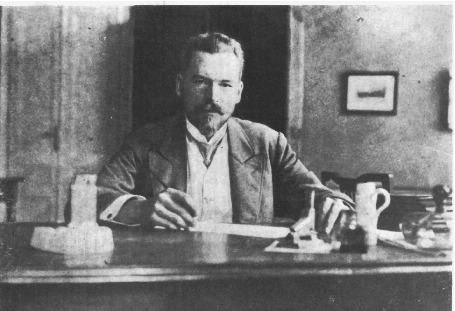#33rd M. Smoluchowski Symposium on Statistical Physics

3-4 December 2020
Europe/Warsaw timezone

## Generalised ‘Arcsine’ laws for run-and-tumble particle in one dimension

3 Dec 2020, 18:24
1m
poster

### Speaker

Mr. Prashant Singh (ICTS-TIFR)

### Description

The ‘Arcsine’ laws of Brownian particles in one dimension describe
distributions of three quantities: the time $t_m$ to reach maximum position, the
time $t_r$ spent on the positive side and the time $t_l$ of the last visit to the
origin. Interestingly, the cumulative distribution of all three quantities are the
same and given by Arcsine function. In this paper, we study distribution of
these three times $t_m$, $t_r$ and $t_l$ in the context of single run-and-tumble particle
in one dimension, which is a simple non-Markovian process. We compute
exact distributions of these three quantities for arbitrary time and find that
all three distributions have a delta function part and a non-delta function
part. Interestingly, we find that the distributions of $t_m$ and tr are identical
(reminiscent of the Brownian particle case) when the initial velocities of the
particle are chosen with equal probability. On the other hand, for $t_l$, only
the non-delta function part is the same as the other two. In addition, we find
explicit expressions of the joint distributions of the maximum displacement
and the time at which this maxima occurs.

### Primary authors

Mr. Prashant Singh (ICTS-TIFR) Prof. Anupam Kundu (ICTS-TIFR)

### Presentation Materials

There are no materials yet.
###### Your browser is out of date!

Update your browser to view this website correctly. Update my browser now

×